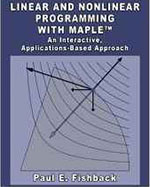Linear and Nonlinear Programming with Maple: An Interactive, Applications-Based Approach - Maplesoft Books - Maple Books, Maple Resources and Math BooksHome : Maplesoft Books : Linear and Nonlinear Programming with Maple: An Interactive, Applications-Based ApproachDetails Search# Linear and Nonlinear Programming with Maple: An Interactive, Applications-Based Approach

## 2009

Description
Integrating a hands-on learning approach, a strong linear algebra focus, Maple™ software, and real-world applications Linear and Nonlinear Programming with Maple™: An Interactive, Applications-Based Approach introduces undergraduate students to the mathematical concepts and principles underlying linear and nonlinear programming. This text fills the gap between management science books lacking mathematical detail and rigor and graduate-level books on mathematical programming.

Throughout the text, topics from a first linear algebra course, such as the invertible matrix theorem, linear independence, transpose properties, and eigenvalues, play a prominent role in the discussion. The book emphasizes partitioned matrices and uses them to describe the simplex algorithm in terms of matrix multiplication. This perspective leads to streamlined approaches for constructing the revised simplex method, developing duality theory, and approaching the process of sensitivity analysis. The book also discusses some intermediate linear algebra topics, including the spectral theorem and matrix norms.

Assuming no prior experience with Maple, the author provides a sufficient amount of instruction for students unfamiliar with the software. He also includes a summary of Maple commands as well as Maple worksheets in the text and online. By using Maple’s symbolic computing components, numeric capabilities, graphical versatility, and intuitive programming structures, students will acquire a deep conceptual understanding of major mathematical programming principles, along with the ability to solve moderately sized real-world applications.

Throughout the book, student understanding is evaluated through "waypoints" that involve basic computations or short questions. Some problems require paper-and-pencil calculations; others involve more lengthy calculations better suited for performing with Maple. Many sections contain exercises that are conceptual in nature and/or involve writing proofs. In addition, six substantial projects in one of the appendices enable students to solve challenging real-world problems.
Categories:
Other Details
Language: English
ISBN: 978-1420090642
Publisher: Chapman and Hall/CRC
 Do you know of a book that should be included in our list? Tell us about it!
 Title/Author: Category: Any Category Abstract Algebra Biology Calculus Chemistry Complex Analysis Computer Algebra Computer Science Conference Proceedings Courseware Cryptography Differential Equations Differential Geometry Dynamical Systems Economics Engineering Games Linear Algebra Maple Manuals Mathematical Modeling Number Theory Numerical Analysis Operations Research Optimization Physics Pre-Calculus Probability & Statistics Programming Recent Releases Vector Calculus Language: Any Language English French German Spanish Japanese Dutch Russian Swedish Polish Persian ChineseAbstract Algebra Biology Calculus Chemistry Complex Analysis Computer Algebra Computer Science Conference Proceedings Courseware Cryptography Differential Equations Differential Geometry Dynamical Systems Economics Engineering Games Linear Algebra Maple Manuals Mathematical Modeling Number Theory Numerical Analysis Operations Research Optimization Physics Pre-Calculus Probability & Statistics Programming Recent Releases Vector Calculus